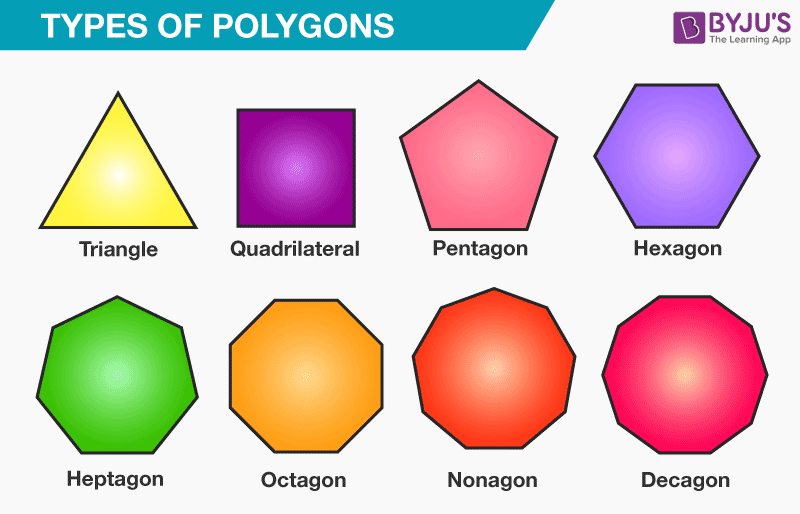# Diagonal Of A Polygon Formula

A polygon is simply a plain figured enclosed by straight lines. In Greek, poly means many and gon means angle. The simplest polygon is a triangle which has 3 sides and 3 angles which sum up to 180 degrees. Here, the diagonal of a polygon formula is given with description and solved examples.There can be numerous sided polygons and they can either be regular (equal length and interior angles) or irregular. A polygon can be further classified as concave or convex based on its interior angles. If the interior angles are less than 180 degrees, the polygon is convex, otherwise, it is a concave polygon. It should be noted the sides of a polygon are always a straight line.

In a polygon, the diagonal is the line segment that joins two non-adjacent vertices. An interesting fact about the diagonals of a polygon is that in concave polygons, at least one diagonal is actually outside the polygon.Now, for an “n” sided-polygon, the number of diagonals can be obtained by the following formula:

Number of Diagonals = n(n-3)/2

This formula is simply formed by the combination of diagonals that each vertex sends to another vertex and then subtracting the total sides. In other words, an n-sided polygon has n-vertices which can be joined with each other in nC2  ways.

Now by subtracting n with nC2  ways, the formula obtained is n(n-3)/2.

For example, in a hexagon, the total sides are 6. So, the total diagonals will be 6(6-3)/2 = 9.Example 1:

Find the total number of diagonals contained in an 11-sided regular polygon.

Solution:

In an 11-sided polygon, total vertices are 11. Now, the 11 vertices can be joined with each other by 11C2 ways i.e. 55 ways.

Now, there are 55 diagonals possible for an 11-sided polygon which includes its sides also. So, subtracting the sides will give the total diagonals contained by the polygon.

So, total diagonals contained within an 11-sided polygon = 55 -11 i.e. 44.

Formula Method:

According to the formula, number of diagonals = n (n-3)/ 2.

So, 11-sided polygon will contain 11(11-3)/2 = 44 diagonals.

Example 2:

In a 20-sided polygon, one vertex does not send any diagonals. Find out how many diagonals does that 20-sided polygon contain.

Solution:

In a 20-sided polygon, the total diagonals are = 20(20-3)/2 = 170.

But, since one vertex does not send any diagonals, the diagonals by that vertex needs to be subtracted from the total number of diagonals.

In a polygon, it is known that each vertex makes (n-3) diagonals. In this polygon, each vertex makes (20-3) = 17 diagonals.

Now, since 1 vertex does not send any diagonal, the total diagonal in this polygon will be (170-17) = 153 diagonals.